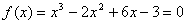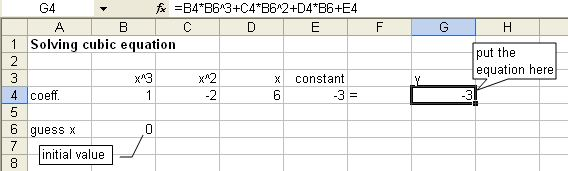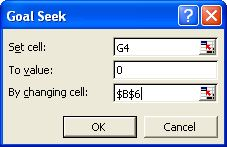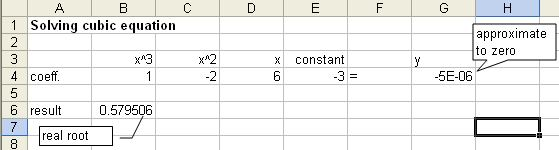## Finding Roots of Polynomial using Microsoft Excel

Let us go down to earth using numerical example, suppose you want to find the real root of this polynomial cubic equationHere is how to do it steps by step:

1. Firstly, input the coefficient of the equations in separate cells (B4:E4).

2. Then, you may guess the value of x with any number as initial value (B6).

3. After that, type the equation of polynomial (G4).

The screen is shown in the figure below4. � From the menu of MS Excel, click Tools-Goal Seek and Goal Seek dialog show up.

5. Set cell G4 ( the equation ) to value 0 ( target value ) by changing cell \$B\$6 ( initial guess of x ) and click OK button.6. The result is shown in the figure below. The real root is 0.5795 when y is approximately zero (-5E-06 = -0.0000050)Note that for other cases, the result may varies depend on the initial condition.

Preferable reference for this tutorial is

Teknomo, Kardi (2015) Finding Root of Polynomial Using Microsoft Excel. https:\\people.revoledu.com\kardi\ tutorial\Excel\RootPolynomial.html